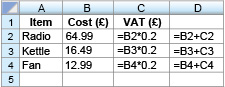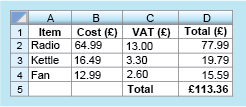Science, Maths & Technology

### Become an OU studentSucceed with maths: part 2

Start this free course now. Just create an account and sign in. Enrol and complete the course for a free statement of participation or digital badge if available.

There is one final activity in this section on spreadsheets, bringing all these ideas together.

Timing: Allow approximately 10 minutes

The formulas used in a spreadsheet are displayed as shown in Figure 3. Note that the asterisk (*) is the notation for multiplication used in spreadsheets and that all entries have been rounded to the closest penny. This example shows UK VAT, which was 20 per cent in 2014 (20 per cent as a decimal is 0.2).• a.The values in columns C and D will be displayed to two decimal places because they represent an amount of money. What values will be displayed in cells C3 and D3?

• a.The formula in C3 is: (to 2 decimal places)

So,

The value in D3 is obtained by adding together the values in B3 and C3.

So,

• b.What do you think is being calculated in the cells in column D? Can you suggest a suitable heading for this column to be entered into D1?

• b.Column D represents the total cost of the item plus VAT. A suitable heading might be ‘Total (£)’. You can think of other correct titles such as ‘Final Price (£)’.
• c.Cell D5 calculates the sum of the values in D2, D3 and D4. Write down a formula that could be entered in cell D5. What does this value represent?